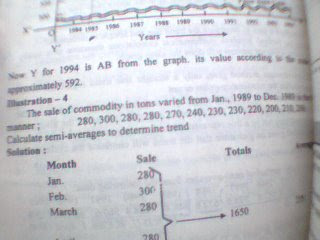Monday, October 13, 2008

Semi Average method2nd method of measuring Trend – Semi Average method

Under this method, we can calculate trend. For this, you have to show your time series on graph paper. For this time is on x’ax and data like sale , production is on y’ax now make original graph by plotting point on graph paper by seeing time and value of data. After plotting original data , now you can calculate trend line . For calculating trend line , you have to calculate semi average . Semi average means average between two time period .

Here are two method of calculating semi average of data which are given below:-

1st method is use when data is even

In this case all time series divides in to two parts and then we calculate average of each part suppose if you have 10 years data then you divide into 5 -5 years half and then you will calculate first five year average and then next five year average after this you have to plot this on the graph paper . This will show the trend line .See picture and understand

2nd method is used when data is odd -

→ in this method you just live middle data and the follow above procedure

Text is available under the Creative Commons Attribution-ShareAlike License; additional terms may apply. By using this site, you agree to our Privacy Policy. Mathematics Education® is a non-profit organization.